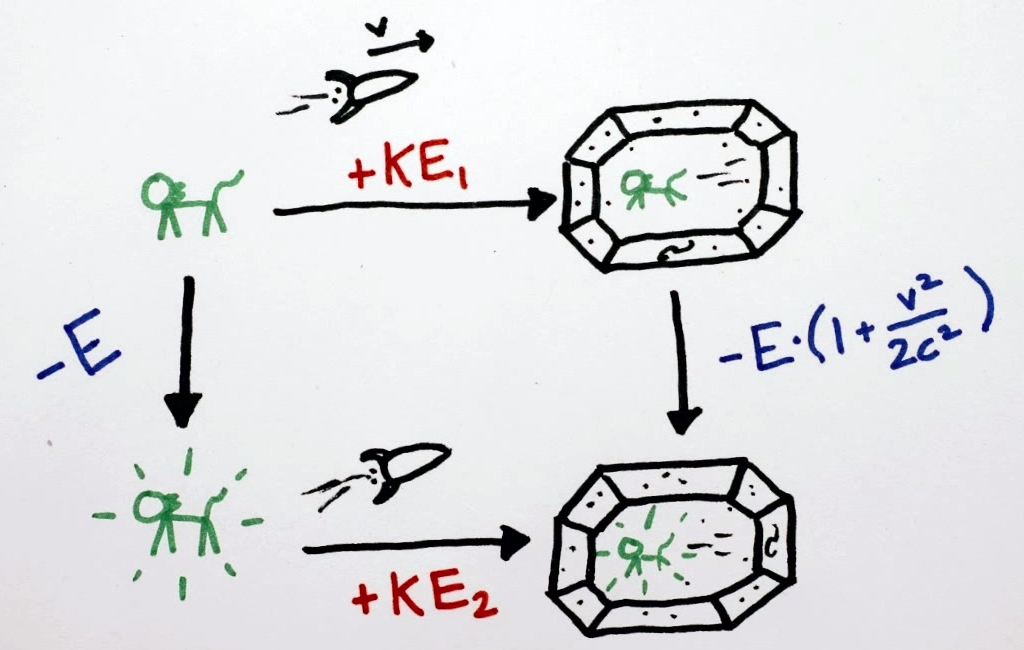E=mc^2 的简单推导

$E = mc^2$

哪里来的能量？1. 经过右上
能量变化为 $$+KE_1 + -E\cdot(1 + \frac{v^2}{2c^2})$$

2. 经过左下
能量变化为 $$-E + KE_2$$

 Tweet